# GMAT Math : DSQ: Calculating the length of a line with distance formula

## Example Questions

### Example Question #1 : Dsq: Calculating The Length Of A Line With Distance Formula

A line segment has an endpoint at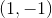; what is its length?

1) Its other endpoint is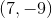2) Its midpoint is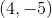BOTH statements TOGETHER are not sufficient to answer the question.
BOTH statements TOGETHER are sufficient to answer the question, but NEITHER statement ALONE is sufficient to answer the question.
Statement 2 ALONE is sufficient to answer the question, but Statement 1 ALONE is not sufficient to answer the question.
EITHER statement ALONE is sufficient to answer the question.
Statement 1 ALONE is sufficient to answer the question, but Statement 2 ALONE is not sufficient to answer the question.
Explanation:

Given the other endpoint, you can use the distance formula to find the length of the segment: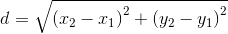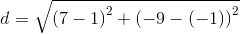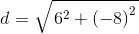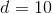Given the midpoint, you can use the distance formula to find the distance from the first endpoint to the midpoint, then double that to get the length of the segment: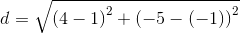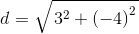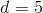The total length is twice that, or 10.

The answer is that either statement alone is sufficient to answer the question.

### Example Question #2 : Dsq: Calculating The Length Of A Line With Distance Formula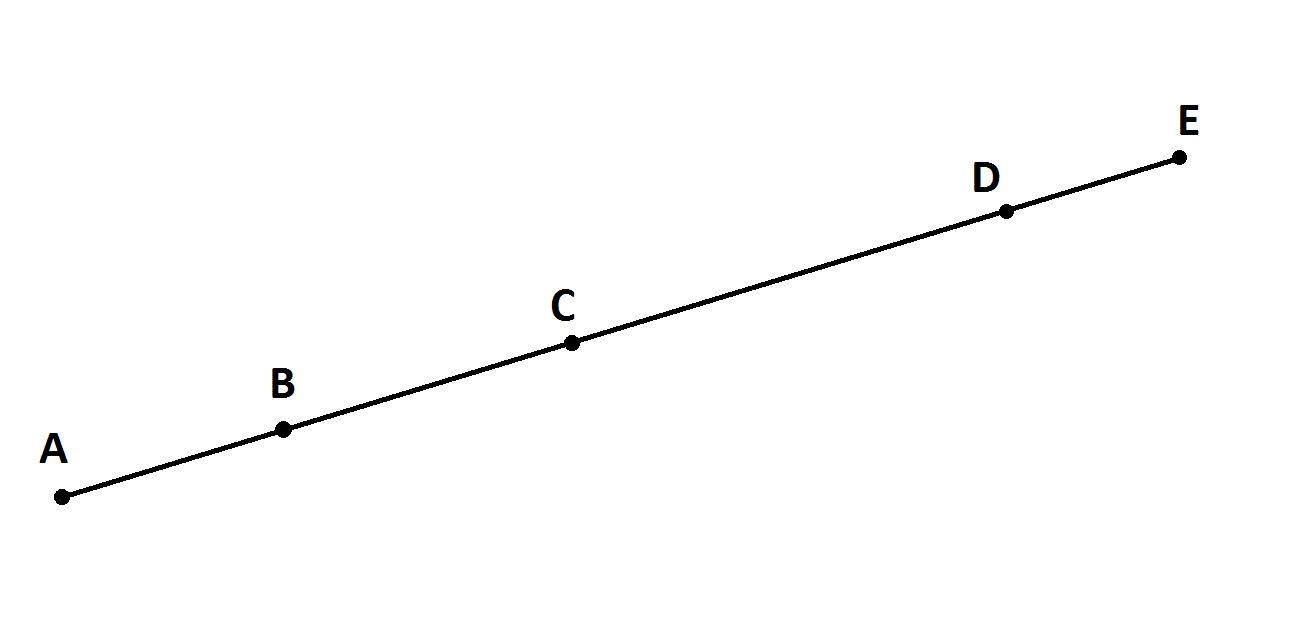Note: Figure NOT drawn to scale.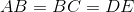Give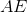.

Statement 1:Statement 2: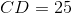Statement 2 ALONE is sufficient to answer the question, but Statement 1 ALONE is NOT sufficient to answer the question.

BOTH statements TOGETHER are sufficient to answer the question, but NEITHER statement ALONE is sufficient to answer the question.

Statement 1 ALONE is sufficient to answer the question, but Statement 2 ALONE is NOT sufficient to answer the question.

BOTH statements TOGETHER are insufficient to answer the question.

EITHER statement ALONE is sufficient to answer the question.

BOTH statements TOGETHER are sufficient to answer the question, but NEITHER statement ALONE is sufficient to answer the question.

Explanation: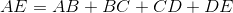If you know only that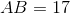, then you know that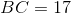and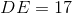, but you still need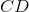, or a way finding it.

If you know only that, you still know only that, but you don't know their actual lengths.

If you know both facts, then you know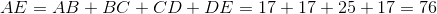### Example Question #3 : Dsq: Calculating The Length Of A Line With Distance Formula

Consider segment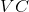.

I) Pointcan be found at the point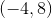.

II) Segmenthad a length ofunits.

Find the coordinates of point.

Either statement alone is sufficient to answer the question.

Statement II is sufficient to answer the question, but statement I is not sufficient to answer the question.

Both statements are necessary to answer the question.

Statement I is sufficient to answer the question, but statement II is not sufficient to answer the question.

Explanation:

Statement I gives us a point.

Statement II gives us the length of the segment.

We are asked to find the coordinates of the other end of the segment. However, we will need more information. Even with all of our information, we have no clue as to the orientation of the line. It could be 14 units straight up and down, it could be a perfectly horizontal line, or something inbetween, thus our answer is:

### Example Question #4 : Dsq: Calculating The Length Of A Line With Distance Formula

Find the length of Segment YZ

I) Point Y is located at the point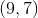.

II) Point Z has a y-coordinate twice that of Point Y and an x-coordinate one-third of Point Y.

Statement I is sufficient to answer the question, but Statement II is not sufficient to answer the question.

Either statement is sufficient to answer the question.

Both statements are needed to answer the question.

Statement II is sufficient to answer the question, but Statement I is not sufficient to answer the question.

Both statements are needed to answer the question.

Explanation:

To find the length of a segment, use the distance formula. The distance formula is given by the following: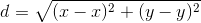Where your's and's correspond to the coordinates of the endpoints.

To find the length of Segment YZ, we need the endpoints.

Statement I gives you Point Y's coordinates.

Statement II relates Point Z's coordinates to Point Y's coordinates. Thus, we can find the point Z using Statement II.

Therefore, we need both.

Recap:

Find the length of Segment YZ

I) Point Y is located at the point.

II) Point Z has a y-coordinate twice that of Point Y, and an x-coordinate one-third of Point Y.

Use Statement II along with Statement I to find the coordinates of Point Z: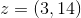Then, use distance formula to find the length of Segment YZ: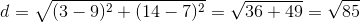### Example Question #5 : Dsq: Calculating The Length Of A Line With Distance Formula

Consider segment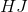I) Endpoint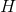is located at the point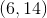.

Ii) Endpoint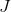has an x-coordinate twice that ofand a y-coordinate 15 times that of H.

What is the length of?

Either statement is sufficient to answer the question.

Statement I is sufficient to answer the question, but statement II is not sufficient to answer the question.

Statement II is sufficient to answer the question, but statement I is not sufficient to answer the question.

Both statements are needed to answer the question.

Both statements are needed to answer the question.

Explanation:

To find the length of a segment, we need both endpoints.

Statement I gives us one endpoint.

Statement II relatesand, allowing us to find the second endpoint.

Thus, we need both. Once we have both endpoints, distance is easily calculated via the distance formula or the Pythagorean theorem.

Using Statement II, we find the second endpoint to be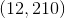. Use the distance formula to find your answer: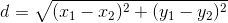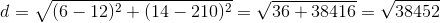Tired of practice problems?

Try live online GMAT prep today.